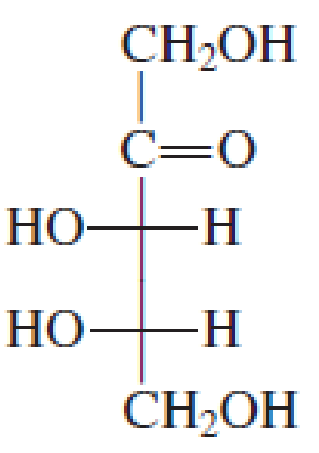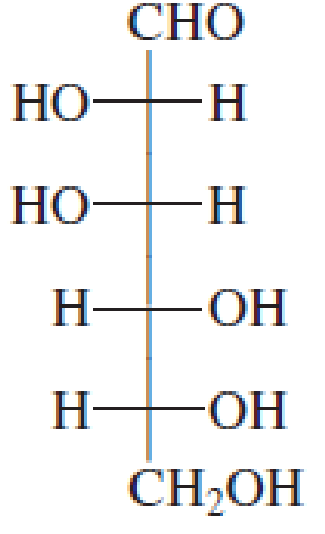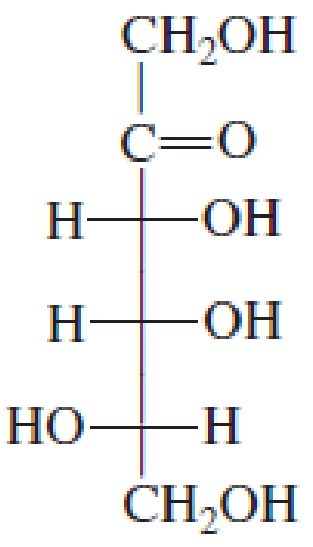Chapter 7, Problem 7.38EP### Organic And Biological Chemistry

7th Edition
STOKER + 1 other
ISBN: 9781305081079

#### Solutions

Chapter
Section### Organic And Biological Chemistry

7th Edition
STOKER + 1 other
ISBN: 9781305081079
Textbook Problem

# Draw a Fischer projection formula for the enantiomer of each of the following monosaccharides.(a)

Interpretation Introduction

Interpretation: The Fischer projection formula for the enantiomer of the given monosaccharide has to be drawn.

Concept introduction: The fischer formula can be sketched by using the following rules:

• • The chiral center is assumed to be in the plane of paper.
• • Two of the bonds are considered pointing towards the page.
• • Two of the bonds are considered pointing outwards the page.
• • The intersection of horizontal and vertical lines represents the chiral center.
• • The horizontal lines are the lines pointing outwards of the page.
Explanation

The given molecule is shown below.

The compounds that form non-superimposable mirror images are called enantiomers...

(b)

Interpretation Introduction

Interpretation: The Fischer projection formula for the enantiomer of the given monosaccharide has to be drawn.

Concept introduction: The fischer formula can be sketched by using the following rules:

• • The chiral center is assumed to be in the plane of paper.
• • Two of the bonds are considered pointing towards the page.
• • Two of the bonds are considered pointing outwards the page.
• • The intersection of horizontal and vertical lines represents the chiral center.
• • The horizontal lines are the lines pointing outwards of the page.

(c)

Interpretation Introduction

Interpretation: The Fischer projection formula for the enantiomer of the given monosaccharide has to be drawn.

Concept introduction: The fischer formula can be sketched by using the following rules:

• • The chiral center is assumed to be in the plane of paper.
• • Two of the bonds are considered pointing towards the page.
• • Two of the bonds are considered pointing outwards the page.
• • The intersection of horizontal and vertical lines represents the chiral center.
• • The horizontal lines are the lines pointing outwards of the page.

(d)

Interpretation Introduction

Interpretation: The Fischer projection formula for the enantiomer of the given monosaccharide has to be drawn.

Concept introduction: The fischer formula can be sketched by using the following rules:

• • The chiral center is assumed to be in the plane of paper.
• • Two of the bonds are considered pointing towards the page.
• • Two of the bonds are considered pointing outwards the page.
• • The intersection of horizontal and vertical lines represents the chiral center.
• • The horizontal lines are the lines pointing outwards of the page.

### Still sussing out bartleby?

Check out a sample textbook solution.

See a sample solution

#### The Solution to Your Study Problems

Bartleby provides explanations to thousands of textbook problems written by our experts, many with advanced degrees!

Get Started